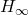A haptic system creates an experience of touch (e.g., force, vibration, and motion) to the user. A vibrating cell phone is an example of a haptic system that we interact with every day. Bilateral teleoperation (i.e., master/slave robotic) system is another interesting example. Such systems let the operator perceive the kinesthetic information that contains the mechanical interactions between the slave robot and its environment. Recent studies show that haptic perception increases the performance of the operator in accomplishing the tasks in terms of applied forces, failures, and completion time.

Our research considers the control design for haptic systems under delayed position and velocity feedback. More precisely, we study the stability of the bilateral teleoperation system. Some second-order ordinary differential equations describe the local mechanical dynamics. The characteristic equation of this system is a quasipolynomial due to the presence of time-delays. A change of variables in the characteristic equation helps to derive the stability conditions analytically. We plot the non-convex region of control gains, where the closed-loop system is asymptotically stable, using Matlab.

Two optimization techniques (and stability margin optimization) choose optimal control gains.optimization minimizes the tracking error between robots while avoiding large control action inputs.analysis requires high computational cost for accurate results due to its dependency on the frequency domain. On the other hand, stability margin optimization defines a cost function to express the trade-off between system bandwidth and robustness with a low computational cost. We applied the results on a real-time experimental platform to illustrate the theoretical results. Finally, we performed a robustness analysis in terms of delay tolerance and parametric uncertainties.

### Publications

 Liacu, B., Koru, A. T., Özbay, H., Niculescu, S. I., Andriot, C. (2013). Optimizing low-order controllers for haptic systems under delayed feedback. Control Engineering Practice, 21(5), 655–668. (link)

 Liacu, B., Koru, A. T., Özbay, H., Niculescu, S. I., and Andriot, C. (2012, June). Low-order controller design for haptic systems under delayed feedback. In 10th IFAC Workshop on Time-Delay Systems (TDS) (pp. 43–48), Boston, MA, USA. (link)

 Koru, A. T. (2012). Controller design for haptic systems under delayed position and velocity feedback. M.Sc. Dissertation, Bilkent University. (pdf)

### Awards

Best Student Contribution Award, June 2012
10th IFAC Time-Delay Workshop, Boston, MA, USA